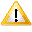# While

(diff) ← Older revision | Latest revision (diff) | Newer revision → (diff)

 LSL Portal

## while( condition ) loop

 • condition – If this executes as true then loop is executed. • loop – Can be either a single statement, a block statement, or a null statement.

Any of the statements can be null statements.

## Specification

Conditional Types
Type Condition
integer True if it is not zero.
float True if it is not zero.
string True if its length is not zero.
key True only if it is a valid key and not NULL_KEY.
vector True if the vector is not ZERO_VECTOR.
rotation True if the rotation is not ZERO_ROTATION.
list True if the length is not zero. Note that correct behavior is only seen with Mono-compiled scripts; LSO-compiled scripts incorrectly resolve to false if the list is non-empty: BUG-230728

## Examples

```//single statement
integer a = 0;
integer b = 10;
while(a < b)
llOwnerSay((string)(a++));
```
```//block statement
integer a = 0;
integer b = 10;
while(a < b)
{
llOwnerSay((string)a);
++a;
}
```
```//null statement
integer a = 0;
integer b = 10;
while(a++ < b);
```Important: Please read this intro of how to iterate over a list in LSL.